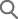• 회원님의 아이디와 패스워드를 입력해 주세요.
• 회원이 아니시면 아래 [회원가입]을 눌러 회원가입을 해주시기 바랍니다.

아이디 저장

HONGGIDONG
사용 가능한 회원 아이디 입니다.

honggildong@naver.com
사용 가능한 E-mail 주소 입니다.

• * 도로명, 건물명, 지번에 대해 통합검색이 가능합니다.
(예: 반포대로 58, 국립중앙박물관, 삼성동 25)
• * 빠른 검색을 위해 주소, 도로명, 건물명, 기타시설물명(지하철역사명/기타시설물 등)을 정확하게 입력하
여 주세요.
• * 약식으로 검색하시는 경우 검색이 제대로 되지 않을 수 있습니다.
(예: 반포, 국중, 삼성)• 아이디/비밀번호를 잊으셨나요? 아래 항목을 정확히 입력해 주시기 바랍니다.

비밀번호 찾기

아이디

성명

E-mail

학술자료 검색

### Structural Breaks or Long Memory for Stock Market Volatility and Volatility Forecasting

In this study we examine whether daily S&P 500 index volatility can be modeled parametrically as a long-memory process by extending an integrated process to a fractionally integrated one. The modified R/S test statistic and others are significant at the 1% level of significance, so we reject the null hypothesis of no long-term dependence. We have found that there is strong evidence for long memory in the series analyzed. We compare the out-of-sample forecasting performance of volatility models from 1962 to 2009. For various forecasting horizons, the long-memory FIGARCH model tends to make more accurate forecasts. Our empirical finding that the index volatility has long memory is consistent with prior evidence showing that an asset market volatility model such as plain GARCH puts too much weight on recent observations in the estimation process relative to those of the past. The forecasting model with the lowest MSFE and VaR forecast error among the models we consider is the FIGARCH model. In terms of forecasting accuracy, it dominates the widely accepted GARCH and rolling window GARCH models. We find that the White’s reality check p-values for the FIGARCH (1, 1) expanding window model reject the hypothesis that there exists a better model than the two benchmark models. The Hansen’s p-values report the same results.
• Hojin Lee
In this study we examine whether daily S&P 500 index volatility can be modeled parametrically as a long-memory process by extending an integrated process to a fractionally integrated one. The modified R/S test statistic and others are significant at the 1% level of significance, so we reject the null hypothesis of no long-term dependence. We have found that there is strong evidence for long memory in the series analyzed. We compare the out-of-sample forecasting performance of volatility models from 1962 to 2009. For various forecasting horizons, the long-memory FIGARCH model tends to make more accurate forecasts. Our empirical finding that the index volatility has long memory is consistent with prior evidence showing that an asset market volatility model such as plain GARCH puts too much weight on recent observations in the estimation process relative to those of the past. The forecasting model with the lowest MSFE and VaR forecast error among the models we consider is the FIGARCH model. In terms of forecasting accuracy, it dominates the widely accepted GARCH and rolling window GARCH models. We find that the White’s reality check p-values for the FIGARCH (1, 1) expanding window model reject the hypothesis that there exists a better model than the two benchmark models. The Hansen’s p-values report the same results.
Conditional Variance; Long Memory; FIGARCH; Structural Break; Out-of-Sample Forecast